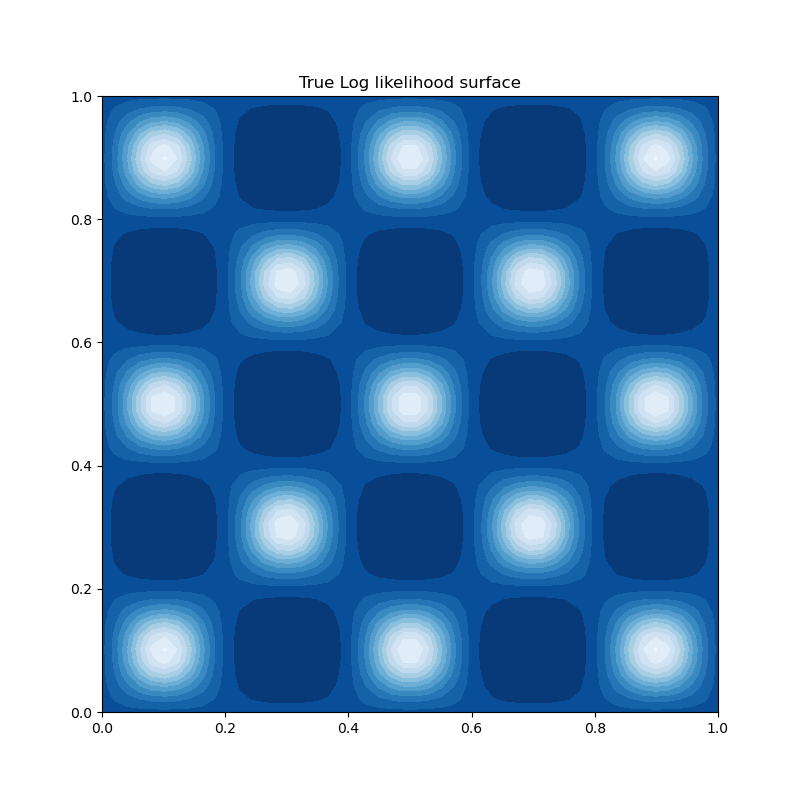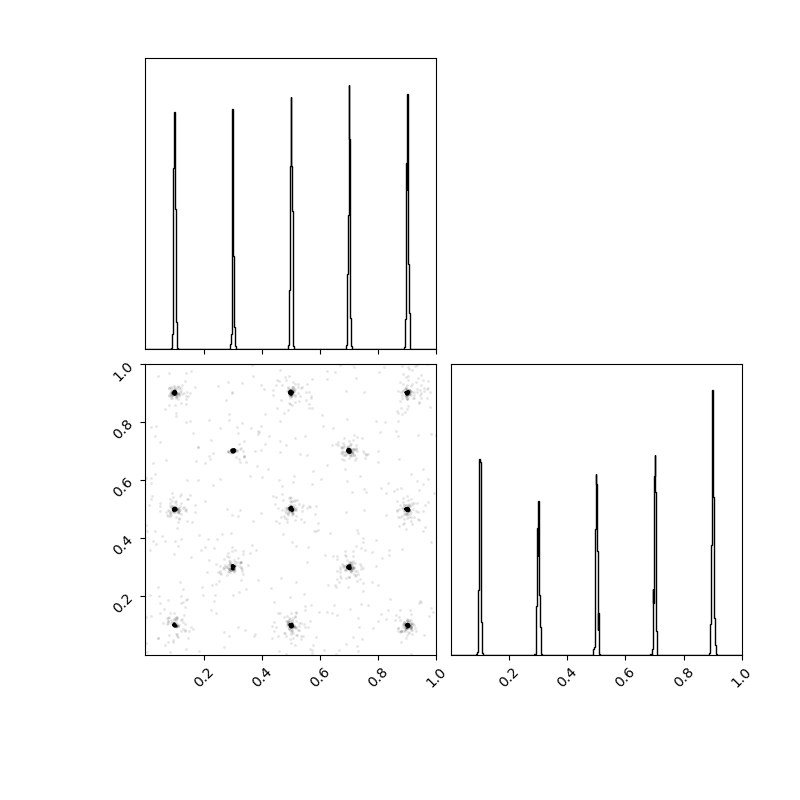# Eggbox¶

A likelihood surface with multiple modes of equal height.

import numpy as np
import matplotlib.pyplot as plt
import corner

import nestle

# Define the posterior density to be sampled:
tmax = 5.0 * np.pi
constant = np.log(1.0 / tmax**2)

def loglike(x):
t = 2.0 * tmax * x - tmax
return (2.0 + np.cos(t/2.0)*np.cos(t/2.0))**5.0

def prior(x):
return x

# plot the surface
plt.figure(figsize=(8., 8.))
ax = plt.axes(aspect=1)
xx, yy = np.meshgrid(np.linspace(0., 1., 50),
np.linspace(0., 1., 50))
Z = loglike(np.array([xx, yy]))
ax.contourf(xx, yy, Z, 12, cmap=plt.cm.Blues_r)
plt.title("True Log likelihood surface")Run nested sampling in multi-ellipsoid mode and print a summary of results:

res = nestle.sample(loglike, prior, 2, npoints=200, method='multi',
update_interval=20)
print(res.summary())


Out:

niter: 1545
ncall: 3035
nsamples: 1745
logz: 236.135 +/-  0.172
h:  5.915


Plot the samples. Note that this represents the likelihood rather than its log, hence it is much more highly peaked.

fig = corner.corner(res.samples, weights=res.weights, bins=500,
range=[(0., 1.), (0., 1.)])
fig.set_size_inches(8., 8.)Total running time of the script: ( 0 minutes 8.239 seconds)

Generated by Sphinx-Gallery